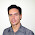## Tuesday, January 2, 2018

### esp8266/Arduino NTC library

A thermistor is a type of negative coefficient resistor whose resistance is dependent on temperature, more so than in standard resistors. The resistance of a NTC Negative Temperature Coefficient thermistor (https://en.wikipedia.org/wiki/Thermistor) decreases as temperature rises. The Steinhart-Hart Thermistor Equation or the Beta Model Equation can be used to correlate the thermistor resistance to temperature to which the sensor is exposed.

It output temperatures in Celsius or Fahrenheit.

The ESP8266 comes with a 10bit ADC, 0 to 1V, one can use the ESP8266 ADC for this library.
Suppose you are going to the internal ADC, and that NTC is going to be used between -20 and +100 degree Celsius. The NTC resistance by datasheet at -20 degree is 100k, and at 100 degree 100. To evaluate the pullup resistor you have to consider that the Voltage output at the ADC pin will be
Vout = Vpullup*(Rntc/Rntc+Rpullup)
We have to consider the max Vout, that comes from the max resistance given by the NTC
Voutmax = Vpullup*Rntcmax/(Rntcmax+Rpullup)
We have to chose a Rpullup such that Voutmax < 1V, so:
Rpullup = Vpullup*Rntcmax/Voutmax - Rntcmax
Subsitute with the sample NTC we are considering:
Rpullup = 3.3*100000/1 - 100000 = 230000.
A 220k resistor would do the job.

In the example i propose here I am using a ADS1015 12 bit analog to digital comparator, that is much more accurate than the internal ESP8266 ADC.
I am using the Adafruit ADS1X15 library for this IC, on top of that library I've build an helper to just convert the ADC raw value that comes from the ADS1x15 to a resistance or voltage value.

Once we've acquired the NTC resistance we can use the Steinhart-Hart Thermistor Equation or the Beta Model Equation to convert the resistance value to temperature.

A digital iir filter is implemented to remove unwanted reading errors.

This library was developed on Atom+PlatformIO, compiled esp8266/Arduino.

Code

Notes

1.did you perchance implement an ADC write method using PWM to simulate. I want to be able to report temp to non IOT things (thermostat/hvac) from GPIO on my ESP8266 to report temperatures from my home sensornet - rather than analog NTC. So an easy way to do this is to 'fake' the NTC temp as an output from the ESP.

1.Hello, sorry for my delay. Unluckly not.

2.Do you have time to help me with instruction where to put these helpers in espeasy firmware ?

1.Hello, unluckly I have no time, I'm fully iunvolved in another project.